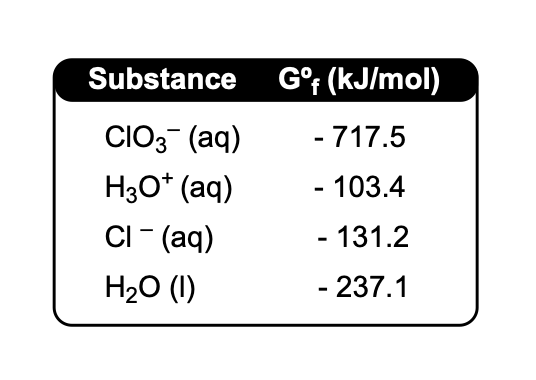Clutch Prep is now a part of Pearson
Ch.18 - ElectrochemistryWorksheetSee all chapters

# Cell Potential and Gibbs Free Energy

See all sections
Sections
Standard Reduction Potentials
Intro to Electrochemical Cells
Galvanic Cell
Electrolytic Cell
Cell Potential: Standard
Cell Potential: The Nernst Equation
Cell Potential and Gibbs Free Energy
Cell Potential and Equilibrium
Cell Potential: G and K
Cell Notation
Electroplating

Cell Potential and Gibbs Free Energy share connections to spontaneity

###### Cell Potential & Gibbs Free Energy

Concept #1: Gibbs Free Energy ties into the capacity of an electrochemical cell to do electrical work.

Example #1: Calculate the maximum electrical work that can be produced by this cell.

3 Co2+ (aq) + 2 Cr (s)  →. 2 Cr3+ (aq) + 3 Co (s)

Given the following reduction potentials:

Cr3+ + 3 e →  Cr                                  E°red = – 0.74 Volts

Co2+ (aq) + 2 e →  Co                        E°red = – 0.28 Volts

Concept #2: Faraday's Constant serves as the bridge betweeen charge and moles of electrons transferred.

Example #2: How many electrons were transferred between an anode and cathode that produced 482.425 kJ of energy?

Practice: What is the gibbs free energy change for the given reaction at 25ºC?

Au3+ (aq) + 3 Li (s)  →. Au (s) + 3 Li+ (aq)

Given the following reduction potentials:

Au3+(aq) + 3 e  →. Au (s)                         E°red = + 1.50 Volts

Li+ (aq) + e  →. Li (s)                                E°red = – 3.04 Volts

Practice: The reduction of chlorate is given by the equation:

ClO3 (aq) + 6 H3O+ (aq)  →  Cl (aq) + 9 H2O (l)

If the standard cell potential is given as 1.373 V, how many electrons are transferred under standard conditions?